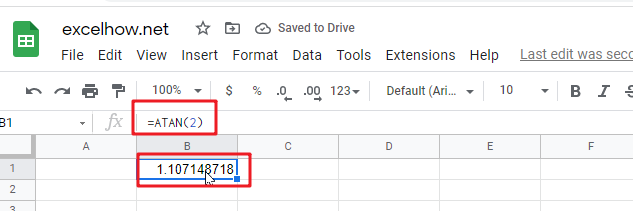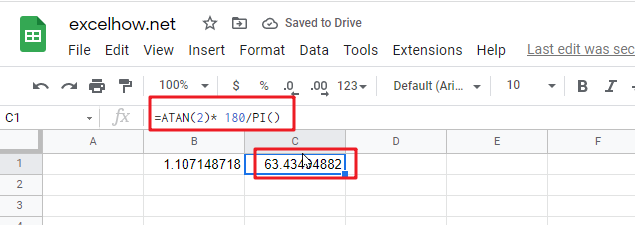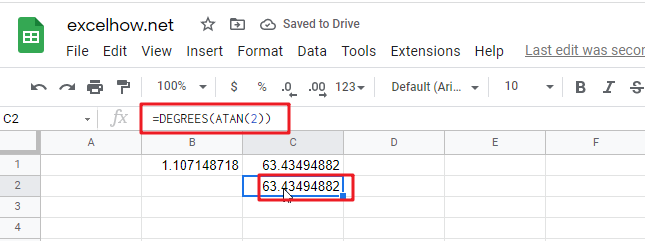# ExcelHow

This post will guide you how to use Google Sheets ATAN function with syntax and examples.

## Description

The Google Sheets ATAN function returns the arctangent of a number. So this function can be used to calculates the arctangent of a supplied number. The returned angle is given in radians in the range –pi/2 to pi/2. Or the returned value is a numeric value.

The purpose of ATAN function is to get arctangent of a given number in google sheets.

The ATAN function is a build-in function in Google Sheets and it is categorized as a MATH function.

## Syntax

The syntax of the ATAN function is as below:

= ATAN (number)

Where the ATAN function arguments is:

• number -This is a required argument. The tangent of the angle that you want to get.

Note:

• If you want to express the arctangent in degrees, you just need to multiply the result by 180/PI() or you can use the DEGREES function.
• If the supplied number is not a numeric value, the ATAN function will get the #VALUE！ Error。

## Google Sheets ATAN Function Examples

The below examples will show you how to use google sheets ATAN Function to return the arctangent for a given number.

1# get the arctangent of 2 in radians, pi/4, enter the following formula in Cell B1.

`=ATAN(2)`The returned result is 1.107149.

2# get the arctangent of 2 in degrees, using the following formula in Cell B2.

`=ATAN(2)* 180/PI()`This formula will return the value 63.43495.

Or you can use the below formula to achieve the same result:

`=DEGREES(ATAN(2))`